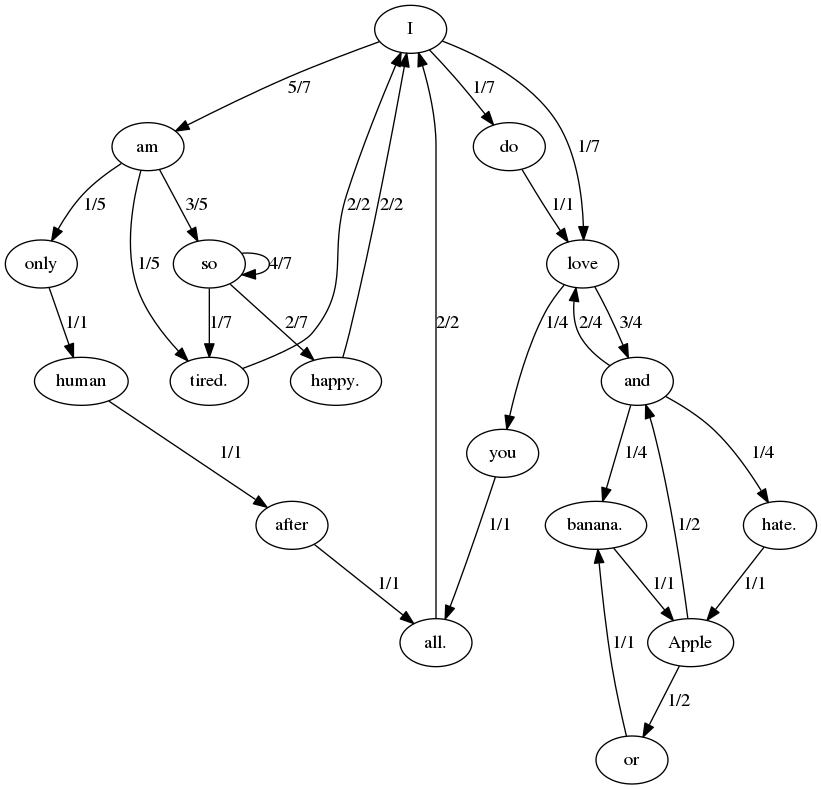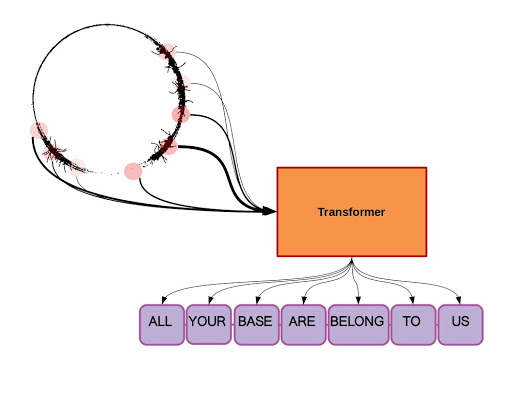# Understanding the Differences in Language Models - Transformers vs. Markov Models

This article explores distinguishing details of Markov Models and Transformer-based models like GPT, focusing on how they predict the next character in a sequence. It explores the fundamental differences between these models, with a particular emphasis on how the self-attention mechanism in Transformer models makes a difference compared to the fixed context length in Markov models.

In the field of machine learning and natural language processing (NLP), different models have been developed to understand and generate human language. Two such models that have gained significant attention are the Markov Models and the Transformer-based models like GPT (Generative Pretrained Transformer). While both types of models can predict the next character in a sequence, they differ significantly in their underlying mechanisms and capabilities. This article aims to delve into the intricacies of these models, with a particular focus on how the self-attention mechanism in Transformer models makes a difference compared to the fixed context length in Markov models.

## Markov Models: A Brief Overview

Markov Models, named after the Russian mathematician Andrey Markov, are a class of models that predict future states based solely on the current state, disregarding all past states. This property is known as the Markov Property, and it is the fundamental assumption that underlies all Markov models.

In the context of language modeling, a Markov Model might predict the next word or character in a sentence based on the current word or character. For instance, given the word "The", a Markov Model might predict that the next word is "cat" based on the probability distribution of words that follow "The" in its training data.

The main limitation of Markov Models is their lack of memory. Since they only consider the current state, they are unable to capture long-term dependencies in a sequence. For example, in the sentence "I grew up in France... I speak fluent ___", a Markov Model might struggle to fill in the blank correctly because the relevant context ("France") is several words back.Figure 1. Markov Model might predict the next word based on the probability distribution of words in its training data. Image Source: markov-chain-text | Modern C++ Markov chain text generator by Jarosław Wiosna

## Transformer Models: An Introduction

Transformer models, on the other hand, were introduced in the seminal paper "Attention is All You Need" by Vaswani et al. (2017). They represent a significant departure from previous sequence-to-sequence models, eschewing recurrent and convolutional layers in favor of self-attention mechanisms.

GPT, developed by OpenAI, is a prominent example of a Transformer model. It is a generative model that can generate human-like text by predicting the next word in a sequence. Unlike Markov Models, GPT considers the entire context of a sequence when making predictions, allowing it to capture long-term dependencies.

## The Power of Self-Attention

The key innovation of Transformer models is the self-attention mechanism. This mechanism allows the model to weigh the importance of different words in the context when predicting the next word. For instance, in the sentence "The cat, which was black and white, jumped over the ___", the model might assign more importance to "cat" and "jumped" when predicting the next word.

Self-attention is calculated using the dot product of the query and key vectors, which are learned representations of the input. The resulting attention scores are then used to weight the value vectors, which are also learned representations of the input. This weighted sum forms the output of the self-attention layer.

The self-attention mechanism allows Transformer models to consider the entire context of a sequence, rather than just the current state. This is a significant advantage over Markov Models, which are limited by their fixed context length.Figure 2. The self-attention mechanism allows Transformer models to consider the entire context of a sequence, rather than just the current state. Image Source: A Deep Dive Into the Transformer Architecture – The Development of Transformer Models by Kevin Hooke

## Fixed Context Length vs. Variable Context Length

Markov Models, due to their inherent design, have a fixed context length. They only consider the current state when making predictions, which limits their ability to capture long-term dependencies. This can lead to less accurate predictions, especially in complex sequences where the relevant context might be several states back.

Transformer models, on the other hand, have a variable context length. Thanks to the self-attention mechanism, they can consider the entire context of a sequence when making predictions. This allows them to capture long-term dependencies and make more accurate predictions.

Moreover, the self-attention mechanism allows Transformer models to dynamically adjust the context length based on the input. For instance, in a sentence with many irrelevant words, the model might focus on a few key words, effectively reducing the context length. This dynamic context length is another advantage of Transformer models over Markov Models.

## Conclusion

While both Markov Models and Transformer models like GPT can predict the next character in a sequence, they differ significantly in their underlying mechanisms and capabilities. Markov Models, with their fixed context length, are limited in their ability to capture long-term dependencies. Transformer models, with their self-attention mechanism, can consider the entire context of a sequence, allowing them to capture long-term dependencies and make more accurate predictions.

Any comments or suggestions? Let me know.

## References

1. Vaswani, A., Shazeer, N., Parmar, N., Uszkoreit, J., Jones, L., Gomez, A. N., ... & Polosukhin, I. (2017). Attention is all you need. In Advances in neural information processing systems (pp. 5998-6008).
2. Radford, A., Wu, J., Child, R., Luan, D., Amodei, D., & Sutskever, I. (2019). Language Models are Unsupervised Multitask Learners. OpenAI Blog.
3. Bishop, C. M. (2006). Pattern Recognition and Machine Learning. Springer.
4. Ruder, S. (2019). The Illustrated Transformer. Jay Alammar's Blog.
5. Brown, T. B., Mann, B., Ryder, N., Subbiah, M., Kaplan, J., Dhariwal, P., ... & Amodei, D. (2020). Language Models are Few-Shot Learners. In Advances in Neural Information Processing Systems.
6. Chollet, F. (2018). Deep Learning with Python. Manning Publications Co.
7. Jurafsky, D., & Martin, J. H. (2019). Speech and Language Processing. Stanford University.
8. Al-Rfou, R., Choe, D., Constant, N., Guo, M., & Jones, L. (2019). Character-Level Language Modeling with Deeper Self-Attention. In Proceedings of the AAAI Conference on Artificial Intelligence.
9. Goodfellow, I., Bengio, Y., & Courville, A. (2016). Deep Learning. MIT press.
10. Manning, C. D., & Schütze, H. (1999). Foundations of Statistical Natural Language Processing. MIT Press.
11. Jurafsky, D., & Martin, J. H. (2009). Speech and Language Processing: An Introduction to Natural Language Processing, Computational Linguistics, and Speech Recognition. Prentice Hall.
12. Jelinek, F. (1997). Statistical Methods for Speech Recognition. MIT Press.
13. Russell, S., & Norvig, P. (2016). Artificial Intelligence: A Modern Approach. Pearson.
14. Charniak, E. (1993). Statistical Language Learning. MIT Press.
15. Lin, T. (2015). Markov Chains and Text Generation. Towards Data Science Blog.
16. Goodman, J. (2001). A bit of progress in language modeling. Microsoft Research.
17. Rosenfeld, R. (2000). Two Decades of Statistical Language Modeling: Where Do We Go From Here?. Proceedings of the IEEE.
18. Nazarko, K. (2021). Word-level text generation using GPT-2, LSTM and Markov Chain. Towards Data Science Blog.
19. Adyatama, A. (2020). Text Generation with Markov Chains Algoritma Technical Blog.

```@article{Saf2023Understanding,